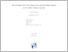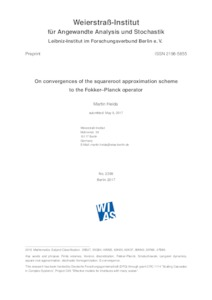Repository: Freie Universität Berlin, Math Department

# Convergences of the squareroot approximation scheme to the Fokker–Planck operator

Heida, M. (2018) Convergences of the squareroot approximation scheme to the Fokker–Planck operator. Mathematical Models and Methods in Applied Sciences, 28 (13). pp. 2599-2635. ISSN 0218-2025, ESSN: 1793-6314Preview

518kB

Official URL: https://dx.doi.org/10.1142/S0218202518500562

## Abstract

We study the qualitative convergence properties of a finite volume scheme that recently was proposed by Lie, Fackeldey and Weber [SIAM Journal on Matrix Analysis and Applications 2013 (34/2)] in the context of conformation dynamics. The scheme was derived from physical principles and is called the squareroot approximation (SQRA) scheme. We show that solutions to the SQRA equation converge to solutions of the Fokker-Planck equation using a discrete notion of G-convergence. Hence the squareroot approximation turns out to be a usefull approximation scheme to the Fokker-Planck equation in high dimensional spaces. As an example, in the special case of stationary Voronoi tessellations we use stochastic two-scale convergence to prove that this setting satisfies the G-convergence property. In particular, the class of tessellations for which the G-convergence result holds is not trivial.

Item Type: Article SFB 1114 Preprint in WIAS Preprint No. 2399: 05/2017 (http://dx.doi.org/10.20347/WIAS.PREPRINT.2399) Mathematical and Computer Sciences > Mathematics > Applied Mathematics 2211 Silvia Hoemke 15 Feb 2018 15:37 04 Jun 2019 16:23

Repository Staff Only: item control page